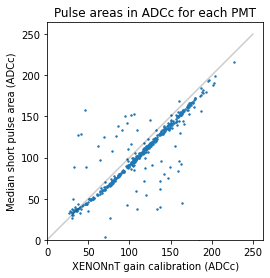# PMT Pulse analysis

Jelle, updated May 2019

updated Feb 2022 by Joran

Standard python setup:

:

import numpy as np
from tqdm import tqdm
import matplotlib.pyplot as plt

# This just ensures some comments in dataframes below display nicely
import pandas as pd
pd.options.display.max_colwidth = 100


After this standard setup, we can load straxen:

:

import strax
import straxen
st = straxen.contexts.xenonnt_online()
straxen.print_versions()

cutax is not installed

:

module version path git
0 python 3.10.0 /home/angevaare/miniconda3/envs/dev_strax/bin/python None
1 strax 1.1.7 /home/angevaare/software/dev_strax/strax/strax branch:master | db14f80
2 straxen 1.2.8 /home/angevaare/software/dev_strax/straxen/straxen branch:master | 024602e

## Raw records

Let’s select a run for which we have strax data.

:

dsets = st.select_runs(available='raw_records',
)
run_id = dsets.name.min()
run_id

:

'008334'

:

st.storage

:

[straxen.storage.rundb.RunDB, readonly: True,
strax.storage.files.DataDirectory, readonly: True, path: /dali/lgrandi/xenonnt/raw, take_only: ('raw_records', 'raw_records_he', 'raw_records_aqmon', 'raw_records_nv', 'raw_records_aqmon_nv', 'raw_records_aux_mv', 'raw_records_mv'),


This run is one hour long, so the full raw waveform data won’t fit into memory. Let’s instead load only the first 30 seconds:

:

rr = st.get_array(run_id, 'raw_records', seconds_range=(0, 30))

convert_channel::       changed channel
convert_channel::       changed channel
convert_channel::       changed channel
convert_channel::       changed channel
convert_channel::       changed channel
convert_channel::       changed channel


The rr object is a numpy record array. This works almost like a pandas dataframe, in particular, you can use the same syntax you’re used to from dataframes for selections.

Here are the fields you can access:

:

st.data_info('raw_records')

:

Field name Data type Comment
0 time int64 Start time since unix epoch [ns]
1 length int32 Length of the interval in samples
2 dt int16 Width of one sample [ns]
3 channel int16 Channel/PMT number
4 pulse_length int32 Length of pulse to which the record belongs (without zero-padding)
5 record_i int16 Fragment number in the pulse
6 baseline int16 Baseline determined by the digitizer (if this is supported)
7 data ('<i2', (110,)) Waveform data in raw ADC counts

You now have a matrix (record_i, sample_j) of waveforms in rr[‘data’]:

:

rr['data'].shape

:

(3046781, 110)


Let’s select only records that belong to short PMT pulses. These are mostly lone PMT hits. Longer pulses are likely part of S1s or S2s.

:

rr = rr[rr['pulse_length'] < 110]


Here’s one record:

:

def plot_record(sample_r):
plt.plot(sample_r['data'][:sample_r['length']], drawstyle='steps-mid')
plt.xlabel("Sample number")

sample_r = rr
plot_record(sample_r)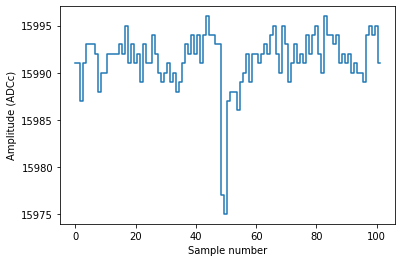As you can see, this record contains a single PE pulse, straight as it comes off the DAQ. No operations have been done on it; we did not even subtract the baseline and flip the pulse.

Each record is 110 samples long, but this digitizer pulse was only 102 samples long:

:

sample_r['pulse_length']

:

102


and thus the pulse has been zero-padded:

:

sample_r['data']

:

array([15991, 15991, 15987, 15991, 15993, 15993, 15993, 15992, 15988,
15990, 15990, 15992, 15992, 15992, 15992, 15993, 15992, 15995,
15991, 15993, 15991, 15992, 15989, 15993, 15991, 15991, 15994,
15992, 15990, 15989, 15990, 15991, 15989, 15990, 15988, 15989,
15991, 15993, 15992, 15994, 15992, 15994, 15991, 15994, 15996,
15994, 15994, 15993, 15993, 15977, 15975, 15987, 15988, 15988,
15986, 15989, 15990, 15992, 15989, 15992, 15992, 15991, 15992,
15993, 15992, 15994, 15995, 15992, 15990, 15995, 15993, 15989,
15991, 15993, 15991, 15992, 15991, 15994, 15992, 15994, 15995,
15992, 15990, 15996, 15994, 15994, 15993, 15994, 15991, 15992,
15991, 15992, 15990, 15991, 15990, 15990, 15989, 15994, 15995,
15994, 15995, 15991,     0,     0,     0,     0,     0,     0,
0,     0], dtype=int16)


Let’s take care of the baseline and zero padding:

:

# Convert from raw_records to the records datatype, which has more fields.
# Without this we cannot baseline.
rr = strax.raw_to_records(rr)

# Subtract baseline and flip channel
strax.baseline(rr)


Now things look more reasonable:

:

plot_record(rr)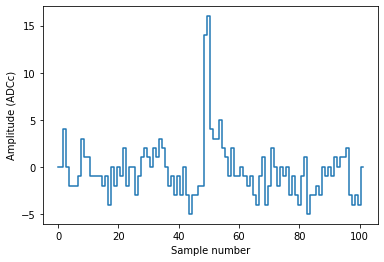## Pulse shape

Let’s focus on channel 100:

:

r = rr[rr['channel'] == 100]


Here is the distribution of amplitudes in each sample. This is very roughly the mean pulse shape:

:

ns = np.arange(len(r['data']))

plt.plot(ns, r['data'].mean(axis=0), drawstyle='steps-mid')
plt.fill_between(
ns,
np.percentile(r['data'], 25, axis=0),
np.percentile(r['data'], 75, axis=0),
step='mid', alpha=0.3, linewidth=0)
plt.xlabel("Sample in record")

:

Text(0, 0.5, 'Amplitude (ADCc)')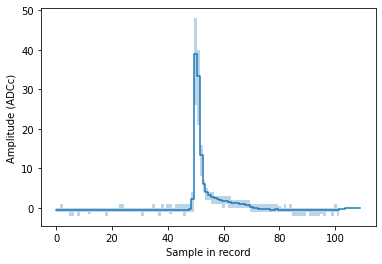You can clearly see we’re dealing with single PEs here, and also see the infamous long PE pulse tail. For a serious pulse shape study you should of course first normalize the pulses.

### Gain calibration

:

run_id

:

'008334'


Let’s integrate between sample 40 and 70, to get the mean single-PE area. We have to add a small correction for the baseline, see here for details. Usually this is done inside strax and you don’t have to worry about it.

:

left, right = 40, 70
areas = r['data'][:,left:right].sum(axis=1)

# Small correction for baseline, see strax issue #2
areas += ((r['baseline'] % 1) * (right - left)).round().astype(np.int64)

# Hack to get ADC -> PE conversion factors. We'll make this better someday...
to_pe = st.get_single_plugin(run_id, 'peaklets').to_pe

plt.hist(areas, bins=100)
plt.ylabel("Counts")
plt.axvline(1/to_pe, color='r', linestyle='--')
plt.axvline(np.median(areas), color='purple', linestyle='--')

:

<matplotlib.lines.Line2D at 0x7fb99a612950>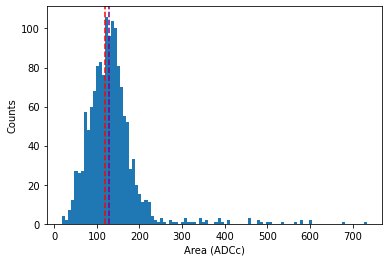The purple line is an extremely bad gain estimate (the median area) of pulses, which nonetheless comes close for this PMT. It should be obvious this is a bad method: it takes no account of 2PE hits or the hitfinder efficiency at all.

The red line indicates where the 1 PE area should be according to the XENON1T gain calibration. Looks like the gain is, at least approximately, correct!

Let’s do the same for all PMTs:

:

areas = rr['data'][:,40:70].sum(axis=1)
channels = rr['channel']

gain_ests = np.array([
np.median(areas[channels == pmt])
for pmt in tqdm(np.arange(straxen.n_tpc_pmts))])

  2%|▏         | 12/494 [00:00<00:16, 29.33it/s]/home/angevaare/miniconda3/envs/dev_strax/lib/python3.10/site-packages/numpy/core/fromnumeric.py:3440: RuntimeWarning: Mean of empty slice.
return _methods._mean(a, axis=axis, dtype=dtype,
/home/angevaare/miniconda3/envs/dev_strax/lib/python3.10/site-packages/numpy/core/_methods.py:189: RuntimeWarning: invalid value encountered in double_scalars
ret = ret.dtype.type(ret / rcount)
100%|██████████| 494/494 [00:18<00:00, 27.23it/s]

:

plt.scatter(1/to_pe, gain_ests, s=2)
plt.plot([0, 250], [0, 250], c='k', alpha=0.2)

/tmp/jobs/17887550/ipykernel_29675/2656599034.py:1: RuntimeWarning: divide by zero encountered in true_divide